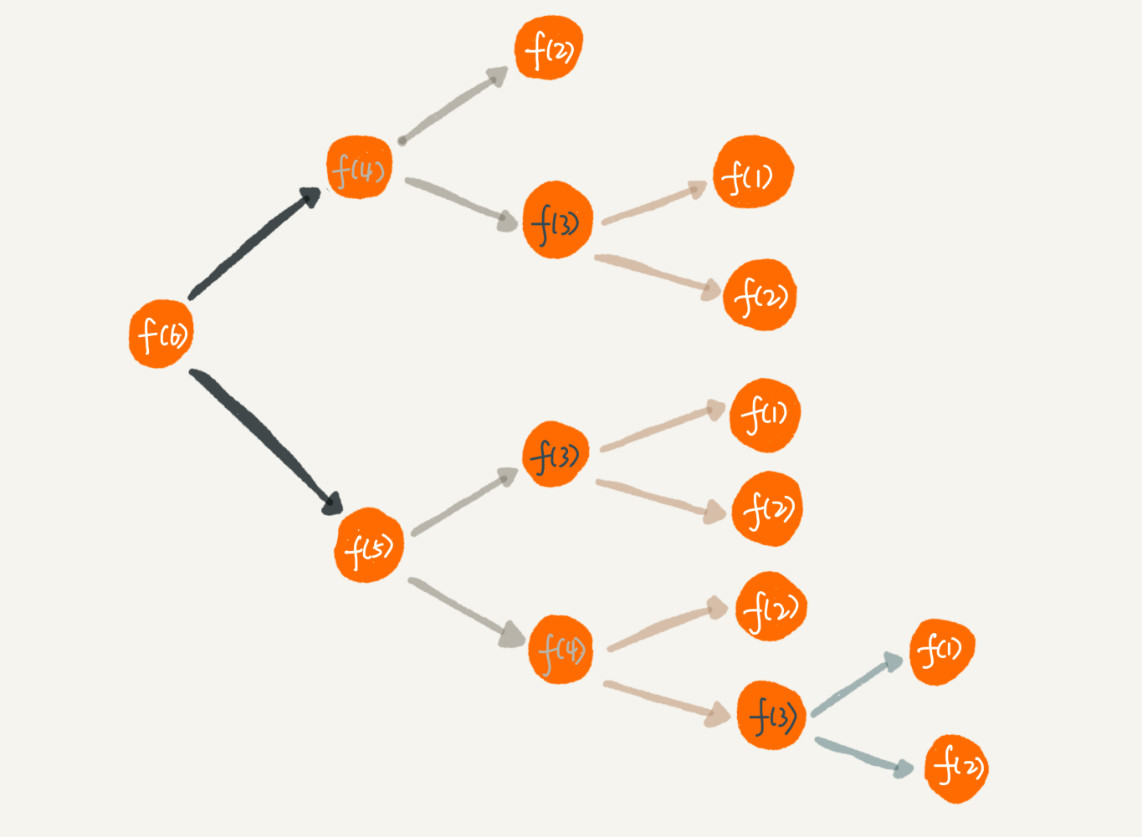## 如何理解“递归”？

``f(n)=f(n-1)+1 其中，f(1)=1``

`f(n)` 表示你想知道自己在哪一排，`f(n-1)` 表示前面一排所在的排数，`f(1)=1` 表示第一排的人知道自己在第一排。有了这个递推公式，就可以很轻松地将它改为递归代码，如下：

``````int f(int n) {
if (n == 1) return 1;
return f(n-1) + 1;
}``````

## 递归需要满足的三个条件

1. 一个问题的解可以分解为几个子问题的解

2. 这个问题与分解之后的子问题，除了数据规模不同，求解思路完全一样

3. 存在递归终止条件

## 如何编写递归代码？

``f(n) = f(n-1)+f(n-2)``

`n=2` 时，`f(2)=f(1)+f(0)`。如果递归终止条件只有一个 `f(1)=1`，那 `f(2)` 就无法求解了。所以除了 `f(1)=1` 这一个递归终止条件外，还要有 `f(0)=1`，表示走 `0` 个台阶有一种走法，不过这样子看起来就不符合正常的逻辑思维了。所以，可以把 `f(2)=2` 作为一种终止条件，表示走 `2` 个台阶，有两种走法，一步走完或者分两步来走。

``````f(1) = 1;
f(2) = 2;
f(n) = f(n-1)+f(n-2)``````

``````int f(int n) {
if (n == 1) return 1;
if (n == 2) return 2;
return f(n-1) + f(n-2);
}``````

## 递归代码要警惕堆栈溢出

``Exception in thread "main" java.lang.StackOverflowError``

``````// 全局变量，表示递归的深度。
int depth = 0;

int f(int n) {
++depth；
if (depth > 1000) throw exception;

if (n == 1) return 1;
return f(n-1) + 1;
}``````

## 递归代码要警惕重复计算``````public int f(int n) {
if (n == 1) return 1;
if (n == 2) return 2;

// hasSolvedList 可以理解成一个 Map，key 是 n，value 是 f(n)
if (hasSolvedList.containsKey(n)) {
return hasSovledList.get(n);
}

int ret = f(n-1) + f(n-2);
hasSovledList.put(n, ret);
return ret;
}``````

## 怎么将递归代码改写为非递归代码？

``````int f(int n) {
int ret = 1;
for (int i = 2; i <= n; ++i) {
ret = ret + 1;
}
return ret;
}``````

``````int f(int n) {
if (n == 1) return 1;
if (n == 2) return 2;

int ret = 0;
int pre = 2;
int prepre = 1;
for (int i = 3; i <= n; ++i) {
ret = pre + prepre;
prepre = pre;
pre = ret;
}
return ret;
}``````

## 思考

• 如何找到“最终推荐人”？解决方案是这样的：

``````long findRootReferrerId(long actorId) {
Long referrerId = select referrer_id from [table] where actor_id = actorId;
if (referrerId == null) return actorId;
return findRootReferrerId(referrerId);
}``````

是不是非常简洁？用三行代码就能搞定了，不过在实际项目中，上面的代码并不能工作，为什么呢？这里面有两个问题。

第一，如果递归很深，可能会有堆栈溢出的问题。

第二，如果数据库里存在脏数据，还需要处理由此产生的无限递归问题。比如 `demo` 环境下数据库中，测试工程师为了方便测试，会人为地插入一些数据，就会出现脏数据。如果 `A` 的推荐人是 `B``B` 的推荐人是 `C``C` 的推荐人是 `A`，这样就会发生死循环。

第一个问题，前面已经解答过了，可以用限制递归深度来解决。第二个问题，也可以用限制递归深度来解决。不过，还有一个更高级的处理方法，就是自动检测 `A-B-C-A` 这种“环”的存在。如何来检测环的存在呢？这个暂时不细说，你可以自己思考下。

• 平时调试代码喜欢使用 `IDE` 的单步跟踪功能，像规模比较大、递归层次很深的递归代码，几乎无法使用这种调试方式。对于递归代码，有什么好的调试方法呢？